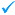150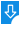10
2585

Calculus 1.0 Description:
Evaluate a function y=f(x) at x=c and estimate limits as x approaches c or x approaches plus or minus infinity. Graph the first derivative f'(x). Graph the second derivative f''(x). Draw the tangent line and calculate the derivative value f'(c) at x=c. Graph the indefinite integral F(x) such that F'(x)=f(x), according to the fundamental theorem of calculus. Calculate the definite integral between x=a and x=b and draw the corresponding area under the curve. Find the arc length of the curve between x=a and x=b. Find the roots x such that f(x)=0. Find maxima and minima of f(x). Find points of inflection of f(x). Compute and draw an approximation to the Taylor series of f(x). Compute and draw an approximation to the Fourier series of f(x). Find a polynomial of degree n-1 passing through n given points using Lagrange interpolation. Find the line of best fit given n points using Gauss' Method of Least Squares. Graph curves using parametric equations. Graph curves using their polar coordinate representation.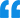Calculus: Graph a function y=f(x).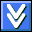Download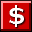BuyScreenshot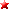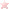Rate it
Category: General Education